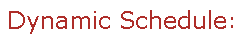Visit Minerva Class Schedule for course dates & times.

# MATH 475 Honours Partial Differential Equations (3 credits)

Offered by: Mathematics and Statistics (Faculty of Science)

### Overview

Mathematics & Statistics (Sci) : First order partial differential equations, geometric theory, classification of second order linear equations, Sturm-Liouville problems, orthogonal functions and Fourier series, eigenfunction expansions, separation of variables for heat, wave and Laplace equations, Green's function methods, uniqueness theorems.

Terms: Fall 2022

Instructors: Choksi, Rustum (Fall)